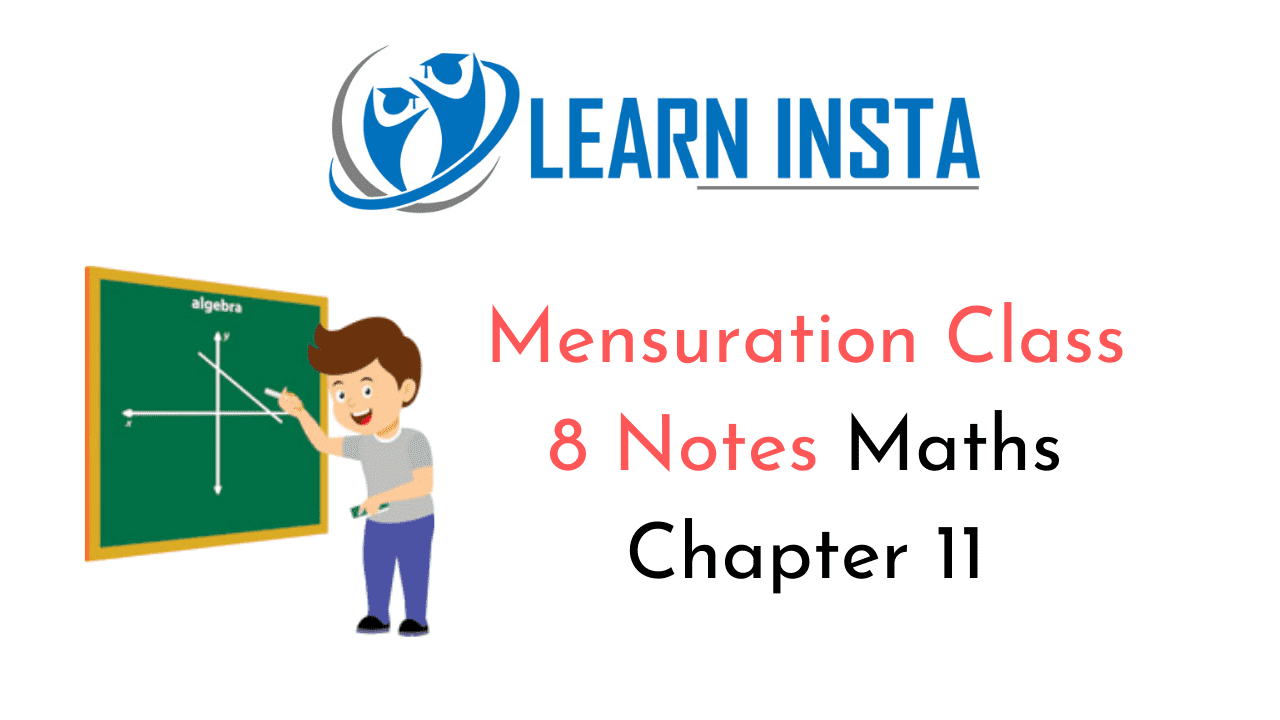On this page, you will find Mensuration Class 8 Notes Maths Chapter 11 Pdf free download. CBSE NCERT Class 8 Maths Notes Chapter 11 Mensuration will seemingly help them to revise the important concepts in less time.

## CBSE Class 8 Maths Chapter 11 Notes Mensuration

### Mensuration Class 8 Notes Conceptual Facts

1. Area of rectangle
= a x b sq. units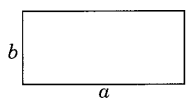2. Area of square
A = (side)2
= a2 sq. units3. Area of triangle
A = $$\frac{1}{2}$$ x b x h sq.units4. Area of equilateral triangle
A = $$\frac{\sqrt{3}}{4} a^{2}$$ sq.units5. Area of parallelogram
A = b x h sq. units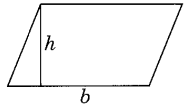6. Area of Circle
A = πr2 sq. units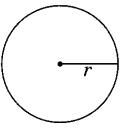7. Area of trapezium
A = $$\frac{1}{2}$$(a + b) x h sq. units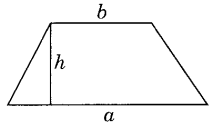A = Area of ΔABC + area of ΔACD
= $$\frac{1}{2}$$ (a + b) x AC sq. units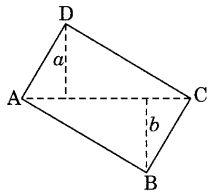9. Area of rhombus
A = $$\frac{1}{2}$$ (d1 x d2) sq. units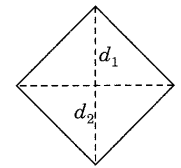10. Surface area of cube
A = 6a2 sq. units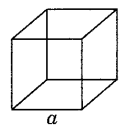11. Surface area of cuboid
A = 2 [ab + bc + ca] sq. units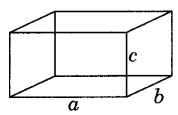12. Surface area of cylinder
A = 2πrh sq. units13. Volume of cube V = a3 units

14. Volume of cuboid V = a x b x c cu. units

15. Volume of cylinder V = πr2h